Courses

# MRP Work Study And Work Measurement - MCQ Test 1

## 20 Questions MCQ Test Mock Test Series - Mechanical Engineering (ME) for GATE 2020 | MRP Work Study And Work Measurement - MCQ Test 1

Description
This mock test of MRP Work Study And Work Measurement - MCQ Test 1 for Mechanical Engineering helps you for every Mechanical Engineering entrance exam. This contains 20 Multiple Choice Questions for Mechanical Engineering MRP Work Study And Work Measurement - MCQ Test 1 (mcq) to study with solutions a complete question bank. The solved questions answers in this MRP Work Study And Work Measurement - MCQ Test 1 quiz give you a good mix of easy questions and tough questions. Mechanical Engineering students definitely take this MRP Work Study And Work Measurement - MCQ Test 1 exercise for a better result in the exam. You can find other MRP Work Study And Work Measurement - MCQ Test 1 extra questions, long questions & short questions for Mechanical Engineering on EduRev as well by searching above.
QUESTION: 1

### In an MRP system, component demand is:

Solution:

In a MRP system, component demand is calculated by the MRP system from the Master Production Schedule.

QUESTION: 2

Solution:
QUESTION: 3

### The proper sequence of activities for material requirement planning is:

Solution:
QUESTION: 4

The individual human variability in time studies to determine the production standards is taken care of by:

Solution:
QUESTION: 5

Match List-I (Files in MRP) with List-II (Inputs required) and select the correct answer: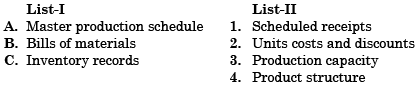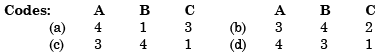Solution:
QUESTION: 6

A welding operation is time-studied during which an operator was pace-rated as 120%. The operator took, on an average, 8 minutes for producing the weld-joint. If a total of 10% allowances are allowed for this operation, the expected standard production rate of the weld-joint (in units per 8 hour day) is:

Solution:

Normal time = 1.2 × 8 = 9.6 minute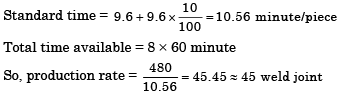QUESTION: 7

Which one of the following is not a necessary information input to Material Requirements Planning?

Solution:
QUESTION: 8

The standard time of an operation while conducting a time study is:

Solution:

Explanation : Thus, Standard time = Average Time × Rating factor + Other allowances.

Standard Time: It is the time, which is taken by a normal worker for a specific task or job, working under moderate conditions and includes other allowances such as fatigue, setting of tool and job, repairing of tool, checking of job etc.

QUESTION: 9

Assertion (A): Master production schedule drives the whole of production and inventory control system in a manufacturing organization.

Reason (R): Master production schedule is a list of daily and weekly work released by PPC to production.

Solution:
QUESTION: 10

Fifty observations of a production operation revealed a mean cycle time of 10 min. The worker was evaluated to be performing at 90% efficiency. Assuming the allowances to be 10% of, the normal time, the standard time (in seconds) for the job is:

Solution:
QUESTION: 11

Which of the following input data are needed for MRP?

1. Master production schedule
2. Inventory position
3. Machine capacity
4. Bill of materials

Select the correct answer using the codes given below:

Solution:
QUESTION: 12

In a time study exercise, the time observed for an activity was 54 seconds. The operator had a performance rating of 120. A personal time allowance of 10% is given. The standard time for the activity, in seconds, is:

Solution: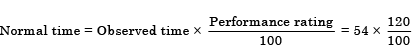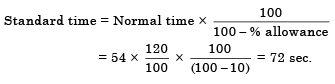QUESTION: 13

Consider the fo llow ing:

1. A master production schedule
2. An inventory status file
3. Bill of material

Which of the above are the inputs to MRP systems?

Solution:
QUESTION: 14

A stop watch time study on an operator with a performance rating of 120 yielded a time of 2 minute. If allowances of 10% of the total available time are to be given, the standard time of the operation is:

Solution:

Standard time of the operation = Basic time + Allowances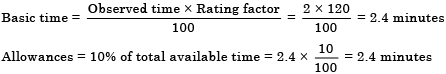Standard time of the operation = 2.4 + 0.24 = 2.64 minutes.

QUESTION: 15

Match List -I (Pro duction con trol function) with List-II (Explanation) and select the correct answer using the code given below the lists: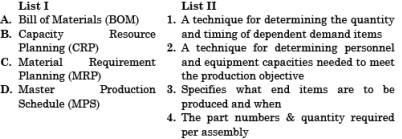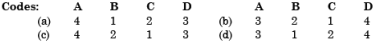Solution:
QUESTION: 16

The actual observed time for an operation was 1 minute per piece. If the performance rating of the operator was 120 and a 5 per cent personal time is to be provided, the standard time in minute per piece is:

Solution: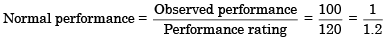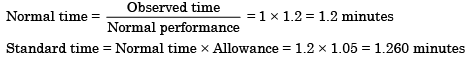QUESTION: 17

Which of the following are needed as the input data for materials requirement planning?

1. Weekly production schedule
2. Bill of material
4. Market forecast

Solution:
QUESTION: 18

A soldering operation was work-sampled over two days (16 hours) during which an employee soldered 108 joints. Actual working time was 90% of the total time and the performance rating was estimated to be 120 percent. If the contract provides allowance of 20 percent of the total time available, the standard time for the operation would be:

Solution: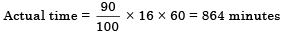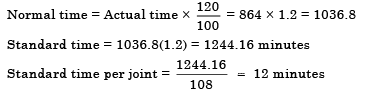QUESTION: 19

Assertion (A): In job design, instead of each job consisting of a single task, a large group of tasks are clustered for a job holder.

Reason (R): A single job should encompass not only production tasks but also the set up, scheduling and control tasks related to the operation.

Solution:
QUESTION: 20

Work study is mainly aimed at

Solution: